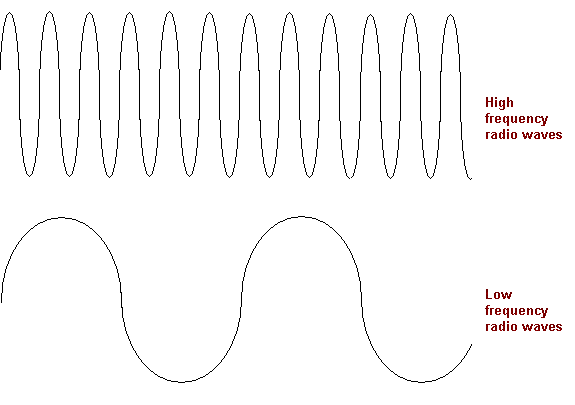## 2008-03-30

### Speed of Sound, Loudness and Amplitude of Sound

Speed of Sound

1. The speed of sound,v, in a medium can be defined as v = fλ, where λ is the wavelength and f is the frequency. The SI units of v is ms-1, f is Hz and λ is  m (metre).

2. The speed of sound in solid is greater than in liquid, and the speed of sound in liquid is greater than in gas.

3. The speed of sound is unaffected by pressure. As an example, iIf the atmospheric pressure changes, the speed of sound in air remains constant.

4. The speed of sound increases with temperature. At the peak of high mountains, the speed of sound is less than that at sea level. This is not due to the lower pressures but because of the lower temperatures at the peak of mountains.

Loudness and amplitude of sound

1. The loudness of sound is considered to be high or low according to the hearing ability of a person.

2. Loudness is influenced by the amplitude of the sound wave.

3. Amplitude has several definitions, according to http://cse.ssl.berkeley.edu/light/measure_amp.html. Amplitude is

• a measurement from the lowest point that the wave hits to the highest point the wave hits.
• a measurement of the top half of the wave.
• a measurement of the distance between two nearest peaks or two nearest troughs.
• a measurement of the bottom half of the wave.Pitch and Frequency of Sound1. The pitch of sound or a musical note is an indication of how high or how low the sound is. Is is a subjective judgement which varies with different individuals.

2. The pitch of a sound is determined by its frequency: a high pitch corresponds to a high frequency.

3. Frequency is how many oscillations a wave complete in a given period of time. Hence you can see that high frequency waves are thinner than low frequency waves because more oscillations are made in the high frequency waves as compared to the low frequency waves within the same period of time.